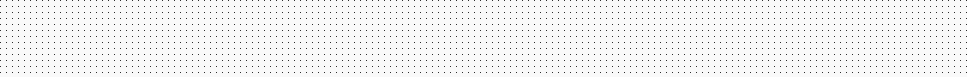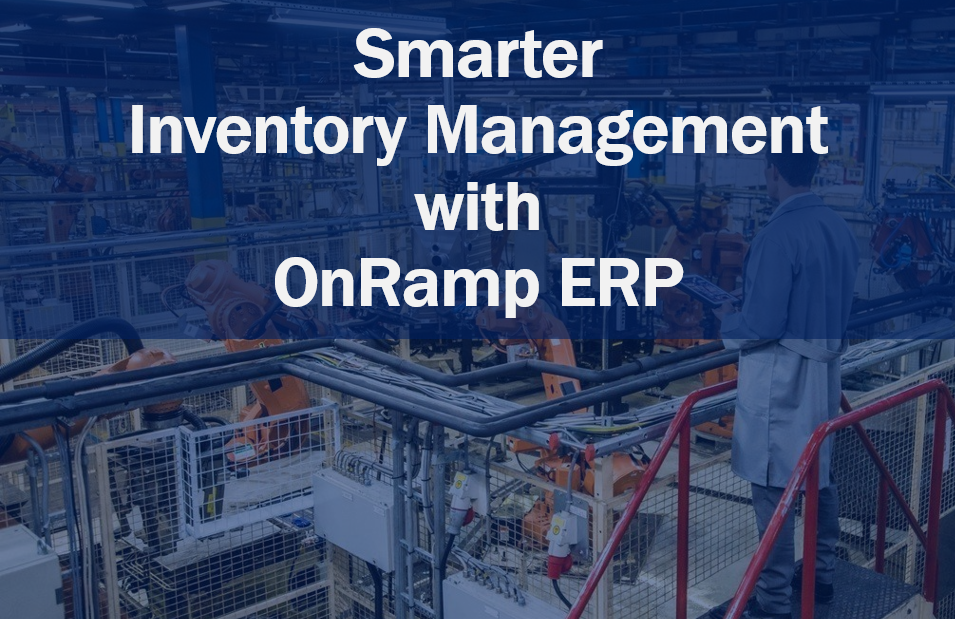# Smarter Inventory Management with OnRamp ERP

A company cannot run LEAN if the amount of inventory it keeps is too high. As mentioned in Going Lean, poor inventory management leads to multiple issues, such as:

• Higher warehouse costs
• Capital tied up with unused inventory
• Unseen defects
• Decreased company response time

Any one of these items is like swimming while holding a boulder for the modern manufactory.

One of the tools that manufacturers use to ensure that they always have enough material on hand while not spending more than needed is Order Policies, or Economic Order Quantity.

As an ERP built from the ground up to work in tandem with LEAN principles, OnRamp ERP has this tool completely integrated into the system.

To help you get started, this post will give you an overview of what Order Policy entails followed by how easy it is to improve your inventory management in OnRamp.

## What is an Order Policy?

An order policy is the company guideline that dictates how many times a year to purchase or manufacture a part in a way that minimizes the total costs. This is also known as the Economic Order Quantity, or EOQ.

A great example of EOQ in practice is presented every time you go to the gas station:

### The Gas Station Conundrum: Fill it up or Only What You Need

When you need gas – how much do you get?

• Wait until the tank is near empty and fill it?
• Fill the tank when the price is low, regardless of the current tank amount?

#### Wait until the tank is near empty

The time taken to stop at the station and refill the vehicle is valuable, i.e., the cost of time is high; whereas the money to purchase the fuel is readily available, i.e., the cost of money is low.

When the “cost” of stopping is higher than the cost of fuel, most people will take this option.

This situation will describe most of our refueling interactions (hush up, you electric car drivers). You fill it up when the needle is near empty, because driving to the station or stopping in the middle of the trip is a hassle. You have places to go and screaming kids that need a nap or a snack. There could be a line-up, a problem at the pump, and the weather could be miserable. And since you always have the money available, the hassle of getting gas is high, but the cost is low.

#### Fill the tank when the price is low

The time taken to stop and refill the vehicle is worth about the same to you as the savings benefit of refilling, i.e., the cost of time and money are equal.

When the “cost” of stopping is equal to the cost of fuel, most people will take this option.

The time taken to stop and refill the vehicle has no measurable worth to you, i.e., the cost of time is low; whereas the money to purchase the fuel is not readily available; i.e., the cost of money is high.

When the “cost” of stopping is lower than the cost of fuel, most people will take this option.

This situation may remind you of your teenage years. When you would add less fuel to the car with each stop, with the needle always on or near empty, because you didn’t have much money. And with lots of time on your hands, the hassle of getting gas was very low.

## EOQ Factors

The EOQ process begins by figuring out:

• How much a part costs to make or buy (Order Cost)
• When buying a part this is the shipping costs.
• When making a part, this is the cost to setup the tool(s).
• How much it costs to have in stock (Carry Cost)
• Carrying costs typically range between 20-30% of a company’s inventory value and represents the cost of the warehouse space and any parts that are lost or damaged while in stock.
• How long it takes to get that part ready (Order Period):
• This is measured in the number of days it takes to get the part from order placed to in stock.

A decision is then made to select the lowest possible total cost value with the highest possible order period for that part.

### Calculating Order Costs

To calculate order cost, you add up all the costs to order the part and multiply that by the number of times the part is ordered. This means that your order costs for a part increase based on the number of times it is ordered. Or as a formula:

Order Cost = Cost To Order x Number of Times Ordered

### Calculating Carry Costs

Carry costs are calculated as a percentage of the Average Inventory (AI). Because of this, calculating the carry costs requires calculating the average inventory first and then multiplying that by your company specified carrying cost, which is usually between 20% – 30%. Or as a formula:

Carry Cost = Average Inventory x Carrying Cost %

#### Average Inventory

Average inventory is the median value of the inventory, based on the number of times the part is order per year. It is calculated by multiplying the part cost by the Annual Volume and dividing by the times ordered per year, then dividing by two. Or as a formula:

Average Inventory = Part Cost x Annual Volume / Times Ordered per Year / 2

### Calculating Order Period

The order period is the number of days between orders. Because of this, to calculate the order period you need to know how many times you order the part per year. Once you know that value, the order period can be found using the following formula:

Order Period = Round Down (No. of Workdays per yr/ No. Orders per yr)

The value used in the number of workdays per year is dependent on the order policy calendar type:

• POQP – This calendar value uses the total number of production days on your shop calendar. For example, for shops that close on the weekends and statutory holidays, in 2019, the total workday value is 261.
• POQC – This calendar value uses the total number of calendar days in the year. For example, in 2019, the total calendar day value is 365.

### Example Order Policy Calculation

This example uses the following values:

• Part Cost = \$60
• Cost to Order = \$20
• Annual Volume = 100 units
• Carrying Cost % = 30%
• Calendar Type = POQP (i.e., no work on weekends or holidays)

#### Order Costs Calculation

• Ordering once per year: \$20 x 1 = \$20/yr
• Ordering once per month: \$20 x 12 = \$240/yr
• Ordering once per week: \$20 x 52 = \$1040/yr

Based on the calculation, ordering once per year resolves to the lowest order costs while ordering weekly is the highest.

#### Carry Cost Calculation

##### Ordering Once per Year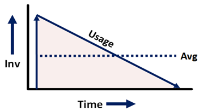Times ordered/yr = 1 Average Inventory = Part Cost x Annual Volume / times ordered per year / 2 Average Inventory = \$60 x 100 / 1 / 2 Average Inventory = \$6000 / 2 Average Inventory = \$3000
##### Ordering Twelve times per Year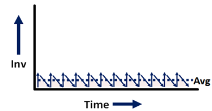Times ordered/yr = 12 Average Inventory = Part Cost x Annual Volume / times ordered per year / 2 Average Inventory = \$60 x 100 / 12 / 2 Average Inventory = \$6000 / 24 Average Inventory = \$250
##### Ordering 52 times per Year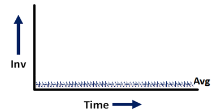Times ordered/yr = 52 Average Inventory = Part Cost x Annual Volume / times ordered per year / 2 Average Inventory = \$60 x 100 / 52 / 2 Average Inventory = \$6000 / 104 Average Inventory = \$57.70

As you can see, the Average Inventory value decreases as you increase the number of times a part is ordered.

• Ordering once per year: \$3000 x 30% = \$900/yr
• Ordering once per month: \$250 x 30% = \$75/yr
• Ordering once per week: \$57.70 x 30% = \$17.31/yr

Based on the calculation, ordering once per year resolves to the highest carry costs while ordering weekly is the lowest.

#### Total Cost Calculation

• Ordering once per year: \$20 x \$900 = \$920
• Ordering once per month: \$240 x \$75 = \$315
• Ordering once per week: \$1040 x \$17.31 = \$1057.31

Based on the calculation, ordering the part once per month, or 12 orders per year, resolves to the lowest order costs.

#### Order Period Calculation

To place 12 orders per year, the order period would be:

• Order Period = Round Down (261/ 12)
• Order Period = Round Down (21.75)
• Order Period = 21

For this order, with an annual amount of 100, OnRamp will suggest that you order 9 parts every 21 production days for a total of 108 parts for the year.

## Economic Order Quantity

OnRamp comes equipped with an EOQ Calculator that provides Order Policy Suggestions that you can use to quickly determine the best order policy for a part.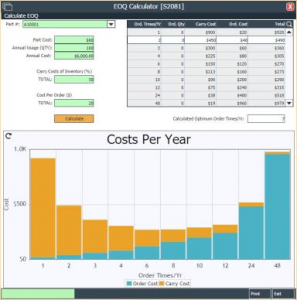The EOQ Calculator provides a graph that displays the total cost per year for different order times per year, with the carry costs displayed in orange, the order costs displayed in blue, and the total cost as the whole bar.

Based on the graph and the table above, you can decide on how much minimization to apply. For example: While the system suggests ordering 7 times per year for the lowest possible total, see Calculated Optimum Order Times/Yr, you may want to increase your order times to 10, since the price difference is \$24 and you would have to carry less inventory, therefore using less space.

## Calculating optimum order times

1. Navigate to the EOQ Calculator [S2081] screen.
2. Select the desired Part.
3. If desired, for fields with a green background, modify the values.
4. Click Calculate.

##### Archives